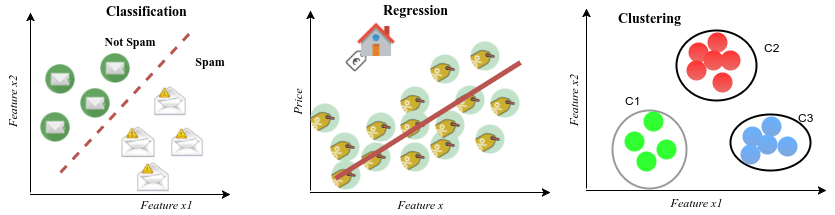##### Classification, Clustering, and Regression, three different perspectives

Nowadays, Artificial Intelligence overtakes other technologies in terms of the capacity of research and investment. But why? Simple but necessary, we need to know what AI methods …

Feb. 21, 2022 4 minuteNowadays, Artificial Intelligence overtakes other technologies in terms of the capacity of research and investment. But why? Simple but necessary, we need to know what AI methods are doing in problems. This will bring a state of perception and let people trust AI.

All AI/ML problems are categorized into three types, classification, clustering, and regression. They are other perceptions and titles in today's complicated world, but most of the concepts can be wrapped up into the above types. You can discover the Supervised, Unsupervised, and Predictive problems, respectively.

Classification is alluding to actions that lead to categorize given examples based on predefined categorizations. On the other hand, clustering algorithm seeks to divide the number of given examples into several categories based on two criteria, high intra-cluster similarity, and low inter-cluster similarity. Regression is utilized to predict an unprecedented example based on the behavior of given examples. These three perspectives toward a problem give us an impression of how to solve a problem.An example will clarify the concepts. Consider a history class with ten students, and we want to classify the students' scores. We predefine the three categories as A (scores between 100-90), B (scores between 90-70), F (scores between below 70), and our machine categorizes the students' scores into these categories. Now consider a Math class with ten students, which we want to cluster the student's scores. Our machine will check the similarity between students' scores, contingent upon the number of clusters (let it be 3), will divide the students' scores into groups. For example, students who got the score 91, 89, 86 into a group, students who got 73, 71, 69, 75, 77, 72 into the second group, and students who got 64, 53 into the third group. A new student will be clustered into one of these clusters simply by calculating the similarity. On the other hand, for regression, our machine considers all the ten students' scores and returns a prediction for the new student who recently joined the class by calculating similar features with the model of the occurred behavior of other students.

Simple as it seems, depending on the problem and the final demand, a type is selected to model even in complex high-dimensional environments. There are methods in these three types to optimize the results more accurately in different contexts and modalities. In Complicated Neural networks adopted for segmentation in an Image Processing task, the method tries to calculate the probability of occurrence of each category and pick the highest possible class. To predict the traffic volume with a given high-dimensional time-series traffic dataset, a clustering method will be adopted.